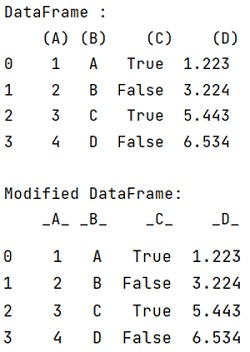# Python - Pandas replace a character in all column names

Given a Pandas DataFrame, we have to replace a character in all column names.
Submitted by Pranit Sharma, on July 30, 2022

Pandas is a special tool that allows us to perform complex manipulations of data effectively and efficiently. Inside pandas, we mostly deal with a dataset in the form of DataFrame. DataFrames are 2-dimensional data structures in pandas. DataFrames consist of rows, columns, and data.

Columns are the different fields that contain their particular values when we create a DataFrame. We can perform certain operations on both rows & column values. Headers are the values assigned to each column, it is an array of values and it acts as a row or headers.

## Replace a character in all column names

Suppose we have three columns in our dataframe named '(A)','(B)' and '(C)' and we need to replace the opening and close parenthesis with _, for this purpose, we will use the following code snippet,

```df.columns.str.replace("[()]", "_")
df[col].str.replace
```

This method is used when we need to replace some string with another string, it returns the updated complete string as a result.

Syntax:

```df['col'].replace('value_to_be_replaced','alternate_value',regex=True)
```

Let us understand with the help of an example,

## Python code to replace a character in all column names

```# Importing pandas package
import pandas as pd

# Creating a dictionary
d = {
'(A)':[1,2,3,4],
'(B)':['A','B','C','D'],
'(C)':[True,False,True,False],
'(D)':[1.223,3.224,5.443,6.534]
}

# Creating a dataframe
df = pd.DataFrame(d)

# Display Dataframe
print("DataFrame :\n",df,"\n")

# Replacing the character in column headers
df.columns = df.columns.str.replace("[()]", "_")

# Display modified DataFrame
print("Modified DataFrame:\n",df)
```

Output:Top MCQs